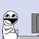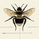# Indicators: KaseCD & Kase Peak Oscillator

9495 visualizzazioni
I have included ports of 2 indicators from MT4 (not from the original commercial source). I couldn't cross check if these matched their commercial equivalents (you need to assume they don't!). If you own any of these in other platforms, appreciate if you could publish some comparison results here.

Kase Peak Oscillator is the difference between two trend measurements, one for rising markets and the other for falling markets. KCD is the PeakOsc minus its own average. So, KPO takes the place of a traditional oscillator and the KCD takes place of the traditional MACD .

Ms .Kase claims KCD is far more accurate than MACD .

- http://www.kaseco.com/support/articles/T...

Let me know if you have experience with the original indicators and are willing to help improve these clones. Thanks.
```//
// @author LazyBear
// If you use this code, in its original or modified form, appreciate if you could
// drop me a note. Thx.
//
study(title="Kase Peak Oscillator [LazyBear]", shorttitle="KPO_LB")
length=input(30, title="Length")
rwh=(high-low[length])/(atr(length)*sqrt(length))
rwl=(high[length]-low)/(atr(length)*sqrt(length))
pk=wma((rwh-rwl),3)
mn=sma(pk,length)
sd=stdev(pk,length)
v1=iff(mn+(1.33*sd)>2.08,mn+(1.33*sd),2.08)
v2=iff(mn-(1.33*sd)<-1.92,mn-(1.33*sd),-1.92)
ln=iff(pk>=0 and pk>0,v1,iff(pk<=0 and pk<0,v2,0))
rbars=iff(pk>pk,pk,0)
gbars=iff(pk>pk,pk,0)
plot(rbars, style=histogram, color=red)
plot(gbars, style=histogram, color=green)
plot(ln, color=yellow, linewidth=1)```
List of my free indicators: http://bit.ly/1LQaPK8
List of my indicators at Appstore: http://blog.tradingview.com/?p=970

## CommentiI see what he means by it being more accurate than the MACD. Thanks for posting this.
RispondiKcd

study(title="Kcd", shorttitle="Kcd")
length=input(30, title="Length")
rwh=(high-low)/(atr(length)*sqrt(length))
rwl=(high-low)/(atr(length)*sqrt(length))
pk=wma((rwh-rwl),3)
kcd=(pk-sma(pk,8))

rbars=iff(kcd>kcd,kcd,0)
gbars=iff(kcd>kcd,kcd,0)
plot(rbars, style=histogram, color=red)
plot(gbars, style=histogram, color=blue)
RispondiCouple interesting ones here LB, not familiar with either, KCD seems to have nice divergence signals
RispondiTheLark
Yup, I have no experience with them either. Not even sure how close this is to the original one :)
Rispondi